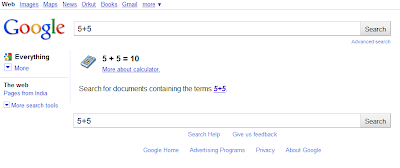How to use google as a calculator? - latest tech tips

Here another very useful but unknown trick from Google. Calculate any mathematical expression in three easy steps.

2. Type in any mathematical expression andGoogle evaluate the result for you.

No need to open any calculator any application nothing simply Google it and you are good.
Below are a few math syntax Google can recognize.
• - for subtraction
• * for multiplication
• / for division
• ^ for exponential (x to the power of y)
• % for modulo (to find the remainder after division)
• choose X choose Y fines the number of possible subset groups of Y out of the set of X.
• th root of creates the nth root of a number
• % of finds percentages X % of Y finds X percent of Y.
• sqrt finds the square root of the number that follows
• ln logarithm base e
• log logarithm base 10
• lg logarithm base 2
• ! factorial – This must follow the number you wish to factor.